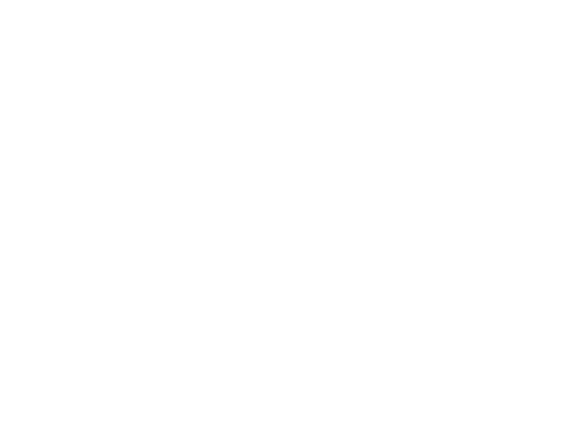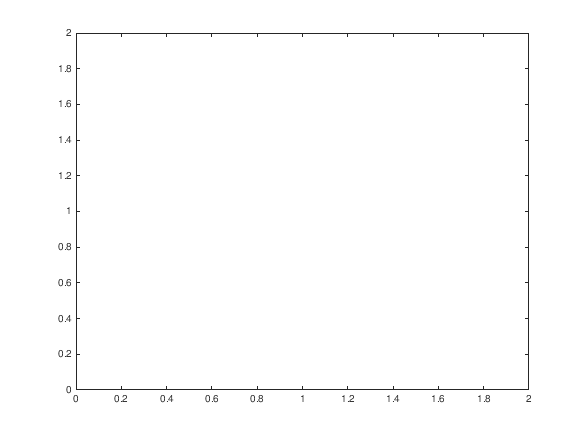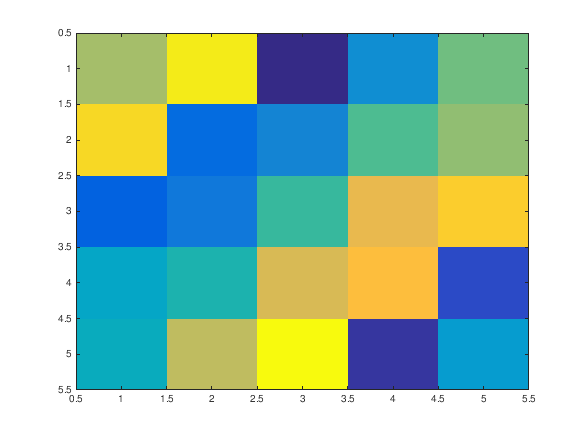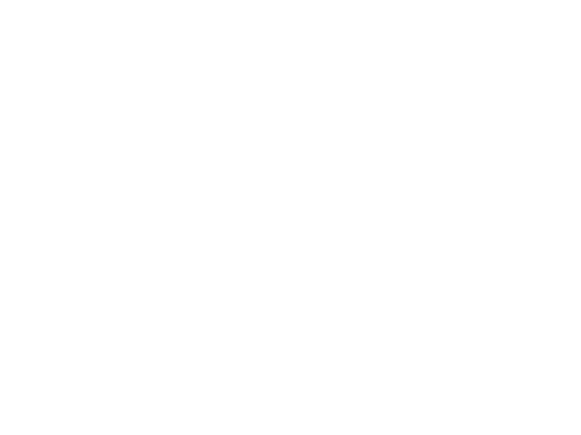Cody

# Problem 43021. How many figures currently exist?

Solution 993394

Submitted on 4 Oct 2016 by Peng Liu
• Size: 12
• This is the leading solution.
This solution is locked. To view this solution, you need to provide a solution of the same size or smaller.

### Test Suite

Test Status Code Input and Output
1   Pass
assert(isequal(numfig(),0))

2   Pass
close all; figure; assert(isequal(numfig(),1))

``
3   Pass
close all; figure; figure; assert(isequal(numfig(),2))

``
``
4   Pass
close all; figure; figure; plot(1,1); figure; imagesc(magic(5)); assert(isequal(numfig(),3))

``
``
``
5   Pass
close all; n = randi(20); for ii=1:n figure; end assert(isequal(numfig(),n))

``
``
``
``
``
``
``
``
``
``
6   Pass
close all; assert(isequal(numfig(),0))

### Community Treasure Hunt

Find the treasures in MATLAB Central and discover how the community can help you!

Start Hunting!